# Digital Simulation of Rubik’s Cube with Qube

This blog post describes Qube, my Rubik's Cube simulator. Source code for Qube is now available in one single file from this link: Qube_osf.m. I will also submit the code to the MATLAB Central File Exchange. As usual, I welcome any feedback.

### Contents

#### Qube

Here is the opening screen for Qube.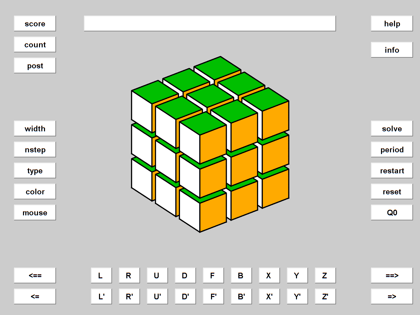#### cube

The cube is displayed in the center of the simulator.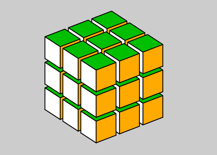#### stack

The current stack is displayed in this text box over the cube.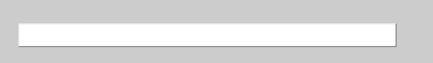#### keys

Eighteen keys use Singmaster notation to generate rotations that are pushed onto the stack.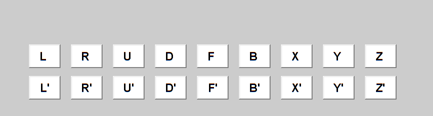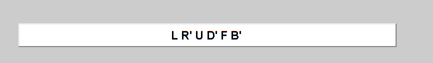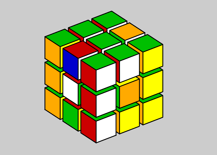#### Scramble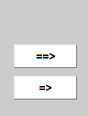The two buttons at the lower right scramble the cube. The => button applies any rotations in the stack to the current cube. To apply them to Q0, use Q0 before =>

The ==> button is a toggle that generates repeated random rotations until it is turned off. A fresh scramble is produced every time I publish this file.

    show_randdo(3,3)
snapnow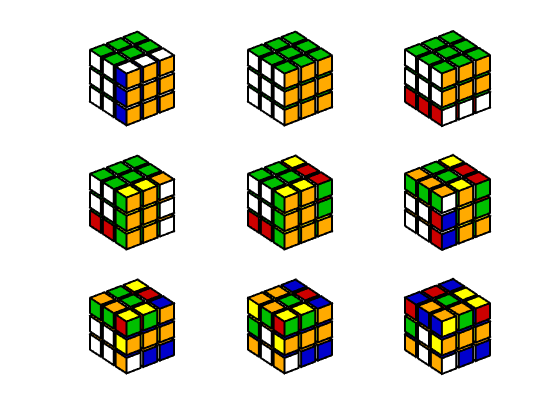#### Unscramble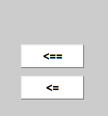The two buttons at the lower left unscramble the cube. The <= button generates a rotation in the direction opposite the rotation at the top of the stack. This acts like a backspace. The <== toggle repeatedly backspaces until the stack is empty. This is the smart way to "solve" the puzzle -- remember how it was scrambled.

Let's unscramble the cube and get back to Q0.

    show_undo(3,3)
snapnow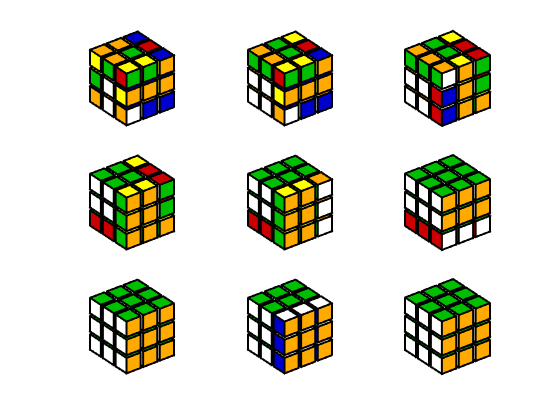#### Appearance

The buttons in the left-hand group control the appearance of the simulator.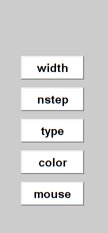width

The vertices of the basic unit cubelet, qzero, are the eight combinations of +1 and -1, so the half-width of qzero is one. The twenty-seven cubelets in Q0 are scaled by this width parameter to produce gaps between the individual cubelets.

    show_width(1,3)
snapnow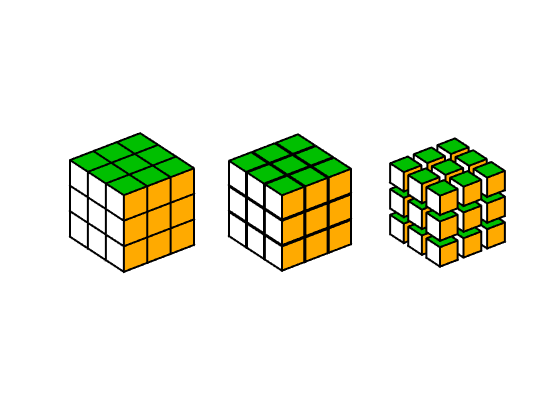nstep

The number of fractional rotations in a quarter turn controls Qube's speed.

type

The cubelet centers are the points [x,y,z] where x, y and z are combinations of -2, 0 and 2. The cubelet type is the number of nonzeros in coordinates of its center, nnz([x,y,z]).

* 0: center
* 1: face
* 2: edge
* 3: corner
    show_types
snapnow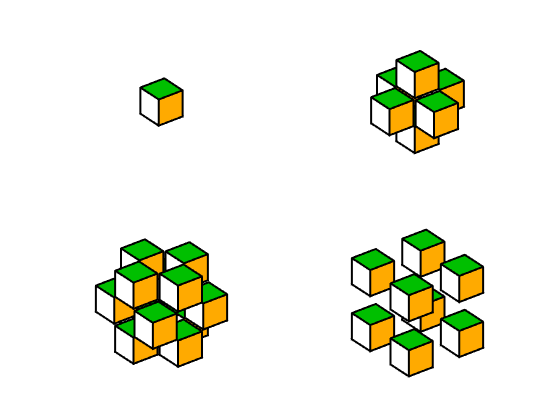color

An alternative to the traditional Rubik's colors made from the MATLAB axes color order.

    show_color(1,3)
snapnow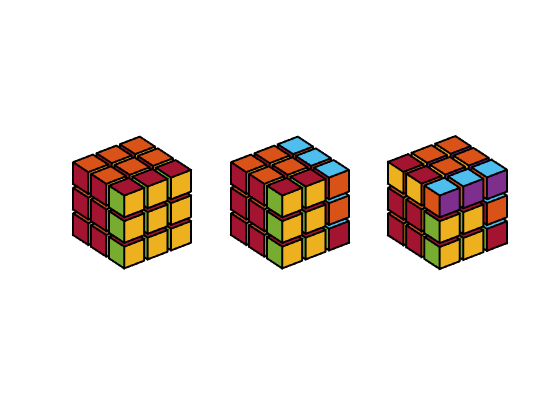mouse

This button turns on Handle Graphics rotate3d. You can then use your mouse to rotate the viewpoint. The visual effect is the same as rotating the entire cube.

#### Actions

The buttons in the right-hand group initiate computations. Other tools may be added.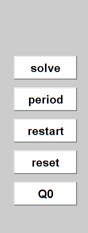solve

Experimental solver. Greedy algorithm to minimize the sum of SVDs of the difference between the cubelets in the current cube and cubelets in Q0. Also known as the nuclear norm. It turns out that this solver does not work very well.

period

Number of repetitions to return to Q0. A fundamental notion in group theory.

reset

Reinitialize without restarting.

restart

Complete restart.

Q0

Restore Q0.

#### Upper left-hand side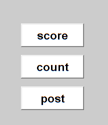score

Currently, the nuclear norm distance to Q0. Need something more sensitive to Rubik's cube patterns.

count

Number of rotations. Hence, the period.

post

Most recent rotation.

#### Upper right-hand side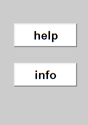help

helpwin Qube

info

Pointer to this blog post.

#### Software

The source code for Qube is available from this link: Qube_osf.m. The osf, one single file, format is a self-extracting archive that expands into a directory of individual functions.

Published with MATLAB® R2022a

|